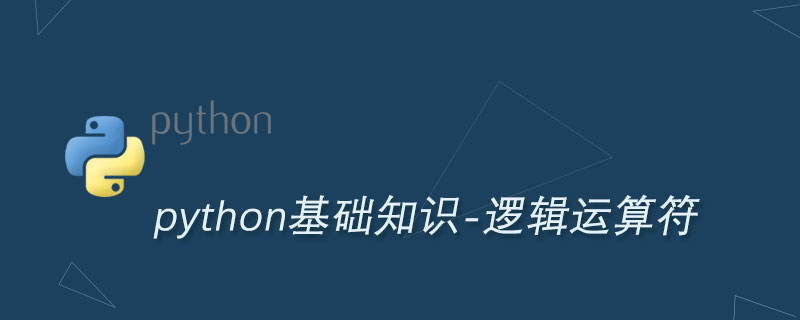# Python逻辑运算符及其用法Python 的逻辑运算符有如下三个：

and：与，前后两个操作数必须都是 True 才返回 True；否则返回 False。

or：或，只要两个操作数中有一个是 True，就可以运回 True；否则返回 False；

not：非，只需要一个操作数，如果操作数为 True，则返回 False；如果操作数为 False，则返回 True。

```# 直接对False求非运算，将返回True
print(not False)
# 5>3返回True，20.0大于10，因此结果返回True
print(5 > 3 and 20.0 > 10)
# 4>=5返回False，"c">"a"返回True。求或后返回True
print(4 >= 5 or "c" > "a")```

```bookName = "C语言中文网Python"
price = 99
version = "正式版"
if bookName.endswith('Python') and price < 50 or version == "正式版" :
print("打算购买这套Python教程")
else:
print("不购买！")```

```bookName = "C语言中文网Python"
price = 99
version = "正式版"
if bookName.endswith('Python') and (price < 50 or version == "正式版") :
print("打算购买这套Python教程")
else:
print("不购买！")```

Python开发开发项目